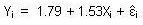### CFA Practice Question

There are 208 practice questions for this study session.

### CFA Practice Question

A regression equation that is derived from ten historical observations is given as follows:If the standard error of the y-intercept is 1.26 and the standard error of the regression coefficient is 0.58, using a 10% significance level, which of the following statements would be incorrect?

A. The lower limit of the estimate for the y-intercept is -0.55, and the upper limit is 4.13.
B. The upper limit of the estimate for the regression coefficient is 1.98.
C. The lower limit of the estimate for the regression coefficient is 0.45.

Use a t-test to define the acceptance range: t0.1/2, 8 = 1.860. Also, the degrees of freedom = n - 2 = 8.

Therefore, the ranges are as follows:
for b0 = 1.79 +/- 1.86 (1.26) = -0.55 to 4.13.
for b1 = 1.53 +/- 1.86 (0.58) = 0.45 to 2.61.

User Comment
Danny20082 The question did not specify the number of observations.
MonkeySee First line:

"A regression equation that is derived from ten historical observations..."
investoprenuer How can it be B? The isn't the upper limit for b1 2.61. Ans B is 1.98
investoprenuer Sorry.... incorrect....
Got it.
shajidubai how did you get 1.98?
Adi8232 lol, chill guys, looks like stress is making your thinking fuzzy.
shash0678 How did we get 1.98?
bablig The queston says which is incorrect...1.98 is INCORRECT.
ashish100 No chill how did they get 1.86?
zagorny95 t table - look for 10% two tail significance level [5% if 1 tail table] for 8 degrees of freedom - Table gives you the result of 1.86